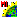# vecop.sml

###Download

#### Syntax Highlighing:

```comments, key words, predefined symbols, class members & methods, functions & classes
```
```# vecop.sml
# Returns output Vector object that is the logical result of two input vectors.
# Possible flag values
# Note, these used to be undocumented numeric flags that you had to add together
# Now they're a string and the Details of Insert Function will tell you what
# values are possible.  If you want to pass more than one, do it like this...
#
#		"TableRemoveDupRec,TableJoinNameStruct"
#
# "RemExRecords"  (Used to be 2)
# "TableJoinNameStruct"  (Used to be 16)
# "TableJoinStruct"  (Used to be 32)
# "TableRemoveDupRec" (Used to be 64)
vector Voperator, Vsource;
vector Vand, Vor, Vreplace, Vsubtract, Vxor;
# get the input vectors
GetInputVector(Voperator);
GetInputVector(Vsource);
# get the output vectors
GetOutputVector(Vand);
GetOutputVector(Vor);
GetOutputVector(Vreplace);
GetOutputVector(Vsubtract);
GetOutputVector(Vxor);
# now create the five output vectors
Vand = VectorAND(Voperator, Vsource);
Vor = VectorOR(Voperator, Vsource);
string flags\$ = "TableRemoveDupRec";
Vreplace = VectorReplace(Voperator, Vsource, flags\$);
Vsubtract = VectorSubtract(Voperator, Vsource, flags\$);
Vxor = VectorXOR(Voperator, Vsource, flags\$);```